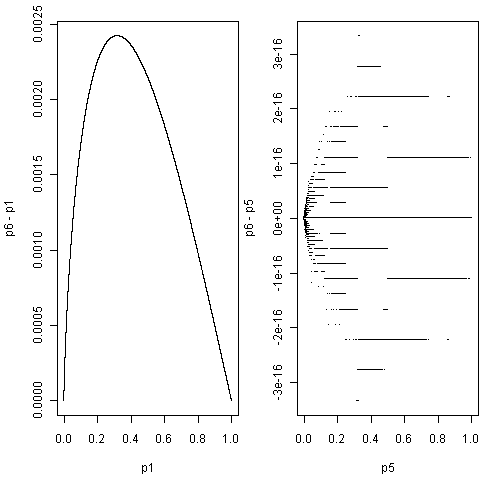## 为了使计算可重复，设定随机数种子
set.seed(123)
## 生成数据，随机数，120000行 x 100列矩阵
dat = matrix(rnorm(120000 * 100), 120000)
nr = nrow(dat)
nc = ncol(dat)
## 六种方法的P值向量
p1 = p2 = p3 = p4 = p5 = p6 = numeric(nr)

## via source()
f = file("test.t")
writeLines(sprintf("p1[%d] = t.test(dat[%d, ])$p.value", seq(nr), seq(nr)), f) system.time({ source(f) }) # user system elapsed # 95.36 0.19 95.86 close(f) unlink("test.t")  # 1、向量式计算：apply()以及*apply() 当我们需要对矩阵的行或者列逐一计算时，apply()系列函数可能会提高效率。本例是对矩阵的行做t检验，那么可以这样计算： ## via apply() system.time({ p2 = apply(dat, 1, function(x) { t.test(x)$p.value
})
})
#   user  system elapsed
#  63.12    0.06   63.50
identical(p1, p2)
#  TRUE


## via for-loop
system.time({
for (i in seq(nr)) p3[i] = t.test(dat[i, ])$p.value }) # user system elapsed # 69.88 0.03 70.05 identical(p2, p3) #  TRUE  不过apply()系列函数在提高运算速度上优势并不会太明显，提倡用它的原因是它和统计中的矩阵运算相似，可以简化代码，相比起$\sum_{i=1}^n x_i/n$，我们可能更愿意看$\bar{x}$这样的表达式。很多R内置函数都是用底层语言写的，我们需要做的就是把一个对象作为整体传给函数去做计算，而不要自行把对象分解为一个个小部分计算，这个例子可能更能体现向量式计算的思想： system.time(apply(dat, 1, mean)) # user system elapsed # 5.28 0.04 5.25 system.time({ x = numeric(nr) for (i in 1:nr) x[i] = mean(dat[i, ]) }) # user system elapsed # 4.44 0.02 4.42 system.time(rowMeans(dat)) # user system elapsed # 0.11 0.00 0.13  # 2、明确计算的目的 很多情况下，R函数返回的不仅仅是一个数字作为结果，而是会得到一系列诸如统计量、P值、各种系数等对象，在我们调用R函数之前如果能想清楚我们究竟需要什么，也许对计算的速度提升有帮助。比如本例中，也许我们仅需要12万个双边P值，其它数字我们都用不着，那么可以考虑“手工”计算P值： ## "hand" calculation in R system.time({ p4 = 2 * pt(apply(dat, 1, function(x, mu = 0) -abs((mean(x) - mu)/sqrt(var(x)/nc))), nc - 1) }) # user system elapsed # 15.97 0.07 16.08 identical(p3, p4) #  TRUE  上面的计算更“纯净”，所以计算速度有了本质的提升，而且计算的结果和前面毫无差异。在做计算之前，人的脑子多思考一分钟，也许计算机的“脑子”会少转一个小时。 # 3、把四则运算交给底层语言 R是高层语言，把它拿来做简单的四则运算是很不划算的，而且容易导致程序低效。加加减减的活儿是C和Fortran等底层语言的强项，所以可以交给它们去做。以下我们用一段C程序来计算t统计量，然后用R CMD SHLIB将它编译为dll（Windows）或so（Linux）文件，并加载到R中，用.C()调用，最终用R函数pt()计算P值： ## "hand" calculation in C for t-statistic writeLines("#include <math.h> void calc_tstat(double *x, int *nr, int *nc, double *mu, double *tstat) { int i, j; double sum = 0.0, sum2 = 0.0, mean, var; for (i = 0; i < *nr; i++) { for (j = 0; j < *nc; j++) { sum += x[i + j * *nr]; } mean = sum / (double) *nc; sum = 0.0; for (j = 0; j < *nc; j++) { sum2 += (x[i + j * *nr] - mean) * (x[i + j * *nr] - mean); } var = sum2 / (double) (*nc - 1); sum2 = 0.0; tstat[i] = (mean - *mu) / sqrt(var / (*nc - 1)); } }", "calc_tstat.c") system("R CMD SHLIB calc_tstat.c") dyn.load(sprintf("calc_tstat%s", .Platform$dynlib.ext))
system.time({
p5 = 2 * pt(-abs(.C("calc_tstat", as.double(dat), nr, nc,
0, double(nrow(dat)))[]), nc - 1)
})
#   user  system elapsed
#   0.86    0.06    0.92
dyn.unload(sprintf("calc_tstat%s", .Platform$dynlib.ext))  因为R可以用system()调用系统命令，所以整个过程全都可以用R完成，Windows用户需要安装Rtools并设置系统环境变量PATH才能使用R CMD SHLIB 更进一步，因为R自身的一些C程序也是可供用户的C程序调用的，所以我们可以把整个P值的计算过程全都扔进C代码中，一步完成： ## "hand" calculation in C calling Rmath.h writeLines("#include <Rmath.h> void calc_pvalue(double *x, int *nr, int *nc, double *mu, double *pval) { int i, j; double sum = 0.0, sum2 = 0.0, mean, var; for (i = 0; i < *nr; i++) { for (j = 0; j < *nc; j++) { sum += x[i + j * *nr]; } mean = sum / (double) *nc; sum = 0.0; for (j = 0; j < *nc; j++) { sum2 += (x[i + j * *nr] - mean) * (x[i + j * *nr] - mean); } var = sum2 / (double) (*nc - 1); sum2 = 0.0; pval[i] = 2 * pt(-fabs((mean - *mu) / sqrt(var / (*nc - 1))), (double) (*nc - 1), 1, 0); } }", "calc_pvalue.c") system("R CMD SHLIB calc_pvalue.c") dyn.load(sprintf("calc_pvalue%s", .Platform$dynlib.ext))
system.time({
p6 = .C("calc_pvalue", as.double(dat), nr, nc, as.double(0),
double(nrow(dat)))[]
})
#   user  system elapsed
#   0.83    0.07    0.91P值的差异

# 小结

1. 若你熟悉底层语言，计算又不太复杂，那么可用底层语言写，然后用R调之；
2. 否则把R对象当做整体去计算，能做x + 1就不要做for (i in length(x)) x[i] + 1
3. 不要低估R core们的编程水平，他们已经做了很多工作让用户脱离底层编程